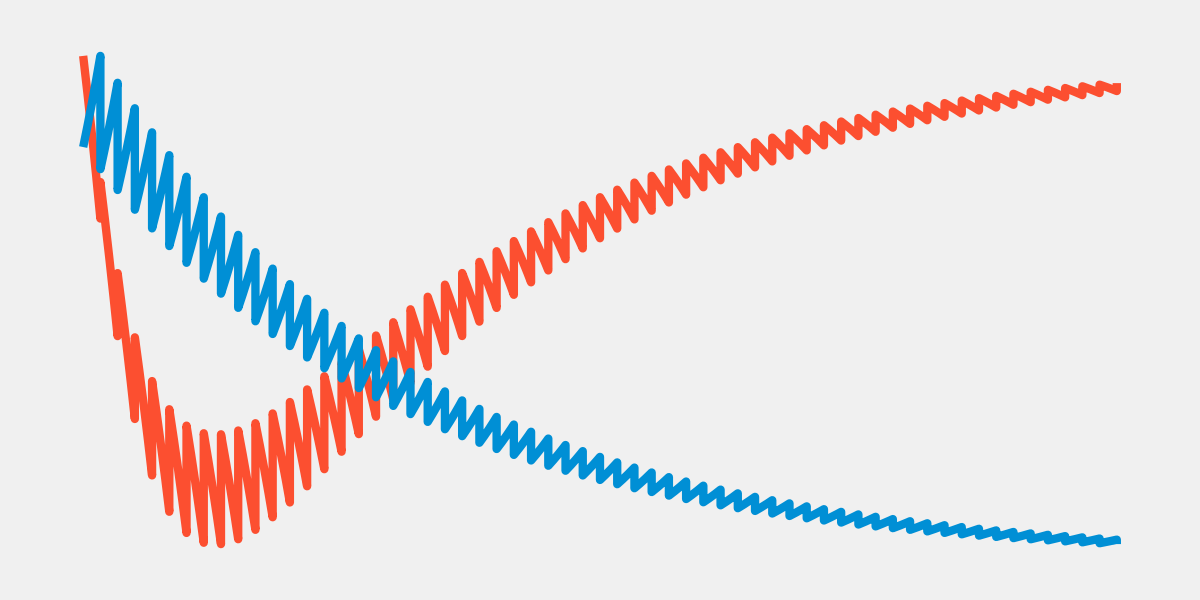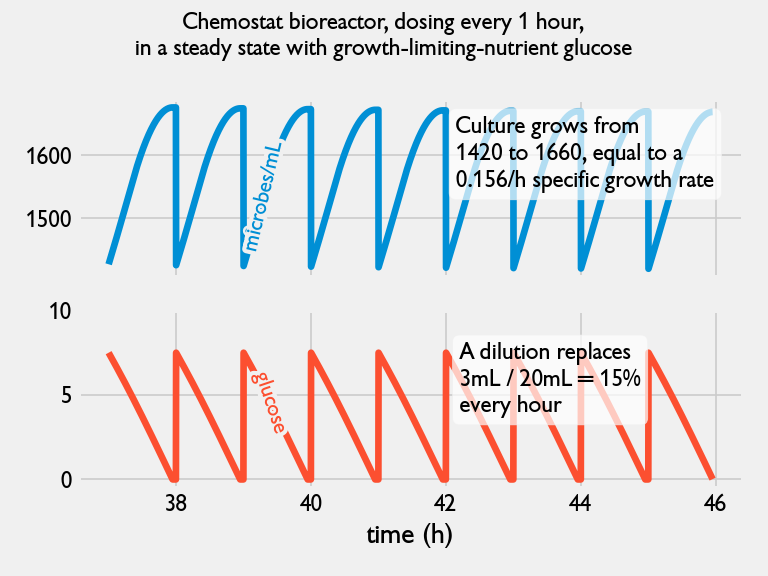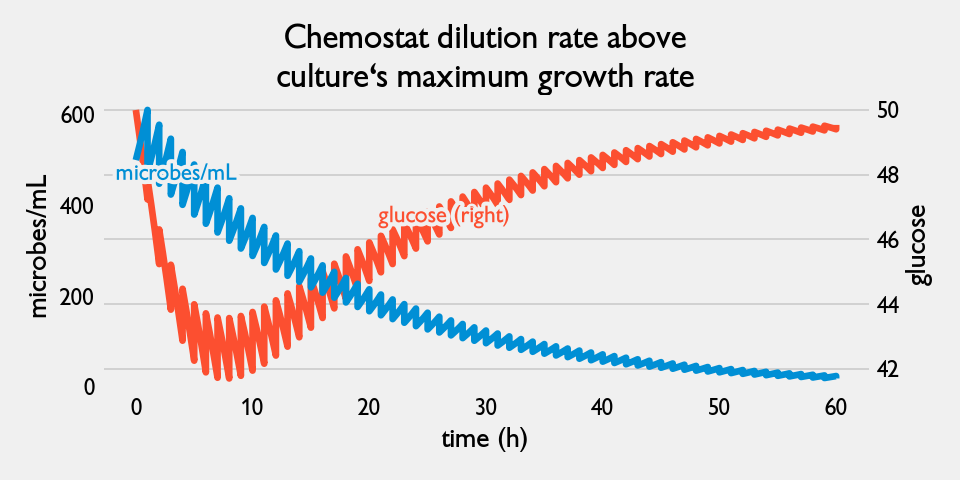# The different strategies of bioreactors: chemostat, turbidostat, stressostat, and more

Bioreactors have an advantage of being able to closely control an environment for microbes. Often, we wish for a bioreactor to precisely control a single, important variable, and we observe how the microbes respond. We can even push this variable to an extreme value - to an environment that the microbes are not familiar with, with interesting results.

When we control a variable in a bioreactor, we often give it a name that ends in stat - short for static. Let's explore the "zoo" of ⋆-stat strategies, what they are used for, how to implement them, and how microbes respond.

### Chemostat

The goal of the chemostat, short for "chemical-static", is to keep all the chemicals in the environment at a near-constant level. This is achieved by continuously adding fresh media and removing an equal volume of old media from the bioreactor.

#### Chemostat strategy

Parameters: period $$P$$ in minutes, volume $$V$$.

Every $$P$$ minutes:
$$\quad$$1. Add  $$V$$ of fresh media to bioreactor
$$\quad$$2. Remove $$V$$ of existing media from bioreactor

One catch is that there will always be a growth rate limiting nutrient that is always nearly depleted - why is this? There is a negative feedback loop between the culture and the rate limiting nutrient: if the rate limiting nutrient is abundant, the culture will grow until the nutrient is depleted. Once the nutrient is depleted, growth stalls until the nutrient is replenished.

This feedback loop ties the growth rate to how fast we replenish the nutrients. For example, if I remove and replace 10% of the media every hour (a dilution), then the remaining culture (of remaining size 91% the original), will grow back to 100%, meaning a 10% growth. In general, in the culture's steady-state, the dilution rate controls the specific growth rate of the culture - in theory, assuming continuous diluting. In practice, there's slight differences if you are diluting periodically.Figure 1. An example of a chemostat in steady state, with glucose as a growth rate limiting nutrient. Units are arbitrary.

In a chemostat, a culture's growth is artificially suppressed, putting an evolutionary pressure to use less of the growth rate limiting nutrient for reproduction. This evolutionary pressure is exploited by bio-engineers to "irrationally" design microbes to require less of a specific nutrient.

The fact that the the chemostat's growth rate is equal to the dilution rate is important for another reason: if the dilution rate is higher than the culture's maximum growth rate, then the culture will be diluted out of the bioreactor.Figure 2. If the dilution rate in a chemostat is set too high, the culture can't keep up and dilutes out.

### Retentostat

While a chemostat will slightly suppress the growth rate of a culture, the retentostat strategy goes to the extreme and forces the growth rate to be 0. It does this by following the chemostat dilution strategy: add fresh media, and remove equal volume of old media but passing the old media through a filter first. This filter keeps the microbes from exiting the bioreactor, that is, causes complete microbe retention ("retention"-stat). So, initially the culture will grow, but will eventually become so dense that all fresh media added will only be used for required microbial upkeep - not for net new growth in the culture. Visually, it looks something like Figure 3.Figure 3. An example of a retenostat. The culture grows until no glucose is available for growing further. Units are arbitrary.

Researchers are interested in zero-growth cultures to understand how their metabolism and -omics differ from a high-growth culture, and to understand cell ageing.

#### Retantostat strategy

Parameters: period $$P$$ in minutes, volume $$V$$.

Every $$P$$ minutes:
$$\quad$$1. Add  $$V$$ of fresh media to bioreactor
$$\quad$$2. Remove $$V$$ of existing media from bioreactor

Similar to a chemostat, there is a very strong selection pressure on the growth yield (i.e. be able to grow more with less resources).

### Turbidostat

The turbidostat's goal is to maintain a constant culture density. The bioreactor uses a turbidity sensor to measure the culture density constantly. When a threshold is reached, a dilution event occurs. If set up correctly (i.e. the threshold is not set too high), then there will not be a growth rate limiting nutrient (because we are artificially suppressing the size of the culture, and so the negative feedback loop is never established). No rate limiting nutrients means that the culture will attain its maximum specific growth rate.

#### Turbidostat strategy

Parameters: volume $$V$$, target optical density $$OD^*$$

Loop for each new optical density signal, $$OD$$:
$$\quad$$If $$OD \ge OD^*$$:
$$\qquad$$1. Add $$V$$ of fresh media to bioreactor
$$\qquad$$2. Remove $$V$$ of existing media from bioreactor

In steady state, the culture will look something like Figure 4 below.Figure 4. An example of a turbidostat in steady state. Units are arbitrary.

There is an evolutionary pressure in a turbidostat culture for a higher maximum growth rate. This can be used to "fix" a genetically engineered organism. Often, after the introduction of a recombinant gene to produce novel proteins or drugs, the microbe's growth rate is lowered (since more energy is spent on production of proteins or drugs, or the culture is inhibited by the protein or drug). There is an opportunity to evolve a higher growth rate by culturing the engineered microbe in a turbidostat - hopefully without modifying the original engineering goal. This is a nice example of "rational design" followed by "irrational design".

### Luminostat

A luminostat, like the name suggests, tries to keep the amount of light constant. This is relevant for photosynthetic cultures which require external light provided by the bioreactor. A light sensor is embedded into the bioreactor, and a control strategy will react to deviations from the a target luminosity. Two options are available: a dilution event will remove microbes from the media, thereby reducing the density and allowing more light to hit the sensor. Alternatively, the bioreactor's lights can increase their lumen output. The former strategy has the added benefit of providing fresh nutrients to the culture, as well.

#### Luminostat strategy 1

Parameters: volume $$V$$, target luminosity $$L^*$$

Loop for each new luminosity signal, $$L$$:
$$\quad$$If $$L \le L^*$$:
$$\qquad$$1. Add $$V$$ of fresh media to bioreactor
$$\qquad$$2. Remove $$V$$ of existing media from bioreactor

#### Luminostat strategy 2

Parameters: target luminosity $$L^*$$

Loop for each new luminosity signal, $$L$$:
$$\quad$$If $$L \le L^*$$:
$$\qquad$$Increase output of LEDs slightly
$$\quad$$Else:
$$\qquad$$Decrease output of LEDs slightly

### Morbidostat

The morbidostat is another strategy to artificially suppress the growth rate of the culture. Instead of using nutrient limitations to suppress the growth rate, a harmful environmental factor is introduced. This factor could be an antibiotic (as in the original morbidostat paper by Toprak ), a significant change in acidity, a change in temperature, etc. Importantly, there is no rate-limiting nutrient due to the repeated dilution with fresh media, like in a turbidostat. This means that there is only a selection pressure on the harmful factor introduced. For example, if I slowly introduce antibiotics without killing the culture, the culture will evolve some resistance to the antibiotic.

In theory, a morbidostat involves two environments: an initial environment where the culture would be happy, and a target environment, where the culture would immediately die if introduced there. The morbidostat slowly introduces the target environment into the initial environment, and allowing the culture to evolve in response. In practice, a morbidostat often has multiple feed systems to add fresh media and fresh media + factor, and an effluent removal system to keep the bioreactor's volume equal.

The introduction of a harmful factor must be balanced against the culture's viability. Too much factor (too much antibiotics, or heat, etc.), can slow the growth rate too much, which slows evolution (I'm reminded of the saying from virology: "no mutation without reproduction"), or it may even kill the culture completely. Using a PID controller to control how much harmful factor to add or remove is useful here. The calculation of growth rate is also very important in a morbidostat, and is usually measured by optical density sensors. The Pioreactor can calculate the growth rate in real time, which allows for much easier and more predictable control over the growth rate. However, approximations to the growth rate can be made using simple calculations with the measured optical density, as in the original paper by Toprak .

#### Morbidostat strategy (high level)

Parameters: target specific growth rate $$R^*$$, tuned PID controller

Loop for each new growth rate measurement, $$R$$:
$$\quad$$If $$R \ge R^*$$:
$$\qquad$$Increase harmful factor in bioreactor, according to the PID controller
$$\quad$$Else:
$$\qquad$$Decrease harmful factor in bioreactor, according to the PID controller

#### Morbidostat strategy (original in )

Parameters: target specific period $$P$$ in minutes, threshold target density $$OD^T$$, volume $$V$$

Every $$P$$ minutes, measure new optical density signal, $$OD$$:
$$\quad$$If $$OD \ge OD^T$$ and $$\Delta OD > 0$$:
$$\qquad$$1. Add $$V$$ fresh media + drug to bioreactor
$$\qquad$$2. Remove $$V$$ existing media from bioreactor
$$\quad$$Else:
$$\qquad$$1. Add $$V$$ fresh media to bioreactor
$$\qquad$$2. Remove $$V$$ existing media from bioreactorFigure 5. A morbidostat evolving antibiotric resistance in E. coli, reproduced from 

### Stressostat

Similar to the morbidostat, the stressostat's goal is to evolve cultures with an end goal in mind. Its design is simpler than the morbidostats but at the cost of only being able to evolve microbes that have a specific behaviour. For example, consider, as the original creators of the stressostat did , the goal of evolving lactic acid bacteria (LAB) to be more lactic acid tolerant. In high concentrations of lactic acid, LAB can grow - albeit quite slowly. The stressostat looks like a chemostat, but instead of diluting with fresh media, the stressostat dilutes with fresh media containing a high concentration of inhibitor - lactic acid in this case. This puts the LAB culture under a constant stress (hence the name). Any mutations that alleviate the stress are self-defeating: the mutant strain will grow, which produces more lactic acid as a byproduct, hence increasing the lactic acid concentration further and putting new stress on the culture. Over time, the final culture will have a much higher lactic acid tolerance than the original culture.

The key behaviour that a culture must have to use the stressostat is that a growing culture creates an inhibitor. LAB cultures have this behaviour, as does yeast cultures. Note that because of the natural negative feedback between the inhibitor concentration and the culture's growth, there's no need for any optical density sensors or growth calculations.

#### Stressostat strategy

Parameters: period $$P$$ in minutes, volume $$V$$.

Every $$P$$ minutes:
$$\quad$$1. Add  $$V$$ of fresh media + high concentration of inhibitor to bioreactor
$$\quad$$2. Remove $$V$$ of existing media from bioreactor

## Conclusion

Well, did I miss any? I'm sure there are more out there. If you'd like to let us know, email us at hello@pioreactor.com and we'll add them.

You may have noticed a pattern in the above strategies: evolution will occur, whether it's desired or not. Whenever the growth rate is suppressed, be it from lack of nutrients, or harmful chemical, or anything else, the culture will respond by trying to skirt around that obstacle. This "escape" can be utilized by engineers, as we saw in some examples above.

The Pioreactor, because of its generality, can handle any of the above bioreactor environments with the right equipment. Read more about how-to's in our Experiments website.

## References

1. Toprak E, Veres A, Yildiz S, Pedraza JM, Chait R, Paulsson J, Kishony R. Building a morbidostat: an automated continuous-culture device for studying bacterial drug resistance under dynamically sustained drug inhibition. Nat Protoc. 2013 Mar;8(3):555-67. doi: 10.1038/nprot.nprot.2013.021. Epub 2013 Feb 21. PMID: 23429717; PMCID: PMC3708598.

2. Sylviani Hartono, Oscar van Mastrigt, Tjakko Abee, Eddy J. Smid. Stressostat: A novel approach in ALE to improve lactate resistance of LAB

3. Vishhvaan Gopalakrishnan, Nikhil P. Krishnan, Erin McClure, Julia Pelesko, Dena Crozier, Kyle J. Card, Drew F.K. Williamson, Nathan Webster, Daniel Nichol, Soumyajit Mandal, Robert A. Bonomo, Jacob G Scott. bioRxiv 729434; doi: https://doi.org/10.1101/729434